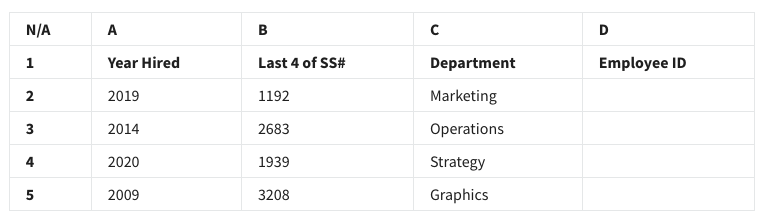## Week 2 – Sparkling-clean data – Shuffle Q/A 2

#### 21. A data analyst in a human resources department is working with the following selection of a spreadsheet:#### They want to create employee identification numbers (IDs) in column D. The IDs should include the last four digits of the employee’s Social Security Number(SS#) plus the year hired. What function will create the ID 32082009 for the employee in row 5?

• =CONCATENATE(B5,A5)
• =CONCATENATE(A5!B5)
• =CONCATENATE(A5+B5)
• =CONCATENATE(B5+A5)

#### 22. Before analyzing a dataset, an analyst maps the data. What is the reason for doing this?

• The analyst wants to know what attributes the data has.
• The analyst thinks the dataset might have some null values.
• The dataset has no visualizations.
• The dataset contains data from different sources.

#### 23. A data analyst suspects that there are many blank cells in their spreadsheet corresponding to missing information. What spreadsheet tool can they use to identify only those cells containing the null values?

• Conditional ranking
• Conditional formatting
• Cell querying
• Cell filtering

#### 24. A data analyst is working on a spreadsheet in which one of the columns is name data. This data is formatted as lastname, firstname. The analyst chooses to divide this data into two new columns, one containing the firstname data and the other containing the lastname data. What spreadsheet tool would they use to do this?

• The MID function
• The SPLIT function
• Substring formatting
• Conditional formatting

• VALUE
• MATCH
• VLOOKUP
• FIND

• =RIGHT(3,C4)
• =LEFT(C4,3)
• =LEFT(3,C4)
• =RIGHT(C4,3)

#### 27. Fill in the blank: In order to make your spreadsheet easier to analyze, you choose to alter the way cells appear if their values meet certain conditions. The spreadsheet tool that you use to do this is called _____.

• conditional formatting
• cell querying
• cell filtering
• conditional ranking

#### 28. An analyst is cleaning a new dataset. They want to make sure the data contained from cell B2 through cell B100 does not contain a number smaller than 10. Which COUNTIF function syntax can be used to answer this question?

• =COUNTIF(B2:B100,”<9″)
• =COUNTIF(B2:B100,”>=10”)
• =COUNTIF(B2:B100,>50)
• =COUNTIF(B2:B200, ”<=50”)

## Shuffle Q/A 2

#### 29. A data analyst is adding new data to the dataset. This data comes from a different source than the current data. What should the data analyst do to ensure the compatibility of the current and new data?

• Use a data visualization
• Map the data
• Spot check for null values
• Apply a data structure

#### 30. A delimiter is a character that indicates the beginning or end of a data item. The split text to columns tool uses a delimiter to accomplish what task?

• To specify where to split a text string
• To split duplicate substrings
• To format a string to numeric
• To change the format of a column of text